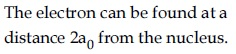#### Which one of the following about an electron occupying the 1s orbitals in a hydrogen atom is incorrect ? ( The Bohr radius is represented by)Option 1)The probability density of finding the electron is maximum at the nucleus.Option 2)The electron can be found at a distancefrom the nucleus.Option 3)The magnitude of the potential energy is double that of its kinetic energy on an average .Option 4)The total energy of the electron is maximum when it is at a distancefrom the nucleus.Total energy of elctron in nth orbit -Where z is atomic number

-

a., so probability density of finding the electron is maximum at the nucleus .

b. True, the electron can be found at any distance from the nucleus.

c.on average.

d.is minimum not maximumis incorrect.

Option 1)

The probability density of finding the electron is maximum at the nucleus.

Option 2)The electron can be found at a distancefrom the nucleus.

Option 3)

The magnitude of the potential energy is double that of its kinetic energy on an average .

Option 4)

The total energy of the electron is maximum when it is at a distancefrom the nucleus.